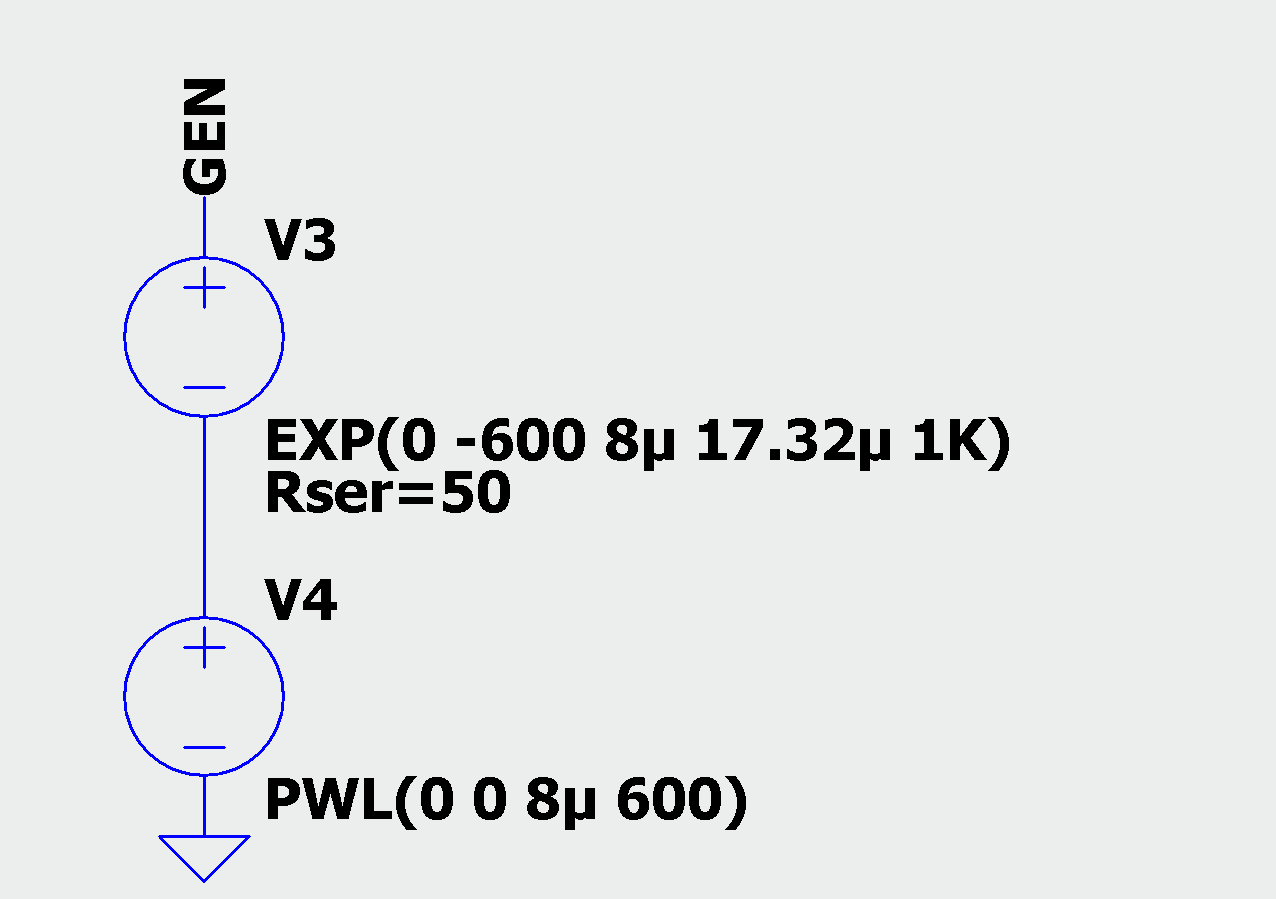LTspice: Using Time-Dependent Exponential Sources to Model Transients

When working with Surge Stoppers or Hot Swap Controllers, occasionally there is a need to simulate a circuit's behavior with a specified voltage or current transient. These transients are usually modeled using a double exponential waveform characterized by a peak voltage, a rise time (usually 10%–90%), a fall time to 50% of the peak voltage and a series resistance.

LTspice® features a double exponential function (EXP) that is ideal for modeling transients via a voltage source. However, it is not as simple as filling in the parameter with tRISE, tFALL and VPEAK. Instead, the EXP function uses standard parameters: VINITIAL, VPULSED, Rise Delay, Fall Delay, Raise Tau, and Fall Tau.

Simple Model for tFall:tRise> 50:1

For waveforms where tFALL : tRISE > 50 : 1 where tFALL is defined from 100%–50% and tRISE is defined from 10%–90%, you can use the following conversions for the EXP function parameters, and under the voltage source’s parasitic properties, enter the appropriate series resistance or add it to the schematic as a separate component:

VINITAL = 0
VPULSED = VPEAK • 1.01
Rise Delay = 0 (for no delay)
Rise Tau = tRISE/2.2
Fall Delay = tRISE
Fall Tau = tFALL • 1.443

Below is an example of a non-repetitive pulse waveform using EXP function with 10μs rise time, 1,000μs fall time, 600V peak and 50Ω series resistance.Sample EXP Voltage Source Settings for 10μs rise time & 1,000μs fall time, 600V peakSample EXP Voltage Source for 10μs Rise Time & 1,000μs Fall Time, 600V Peak

The waveforms below show the results of the above EXP voltage source with an open circuit, VGEN, and clamped with a TVS clamp, VIN. Also shown is the instantaneous power dissipation (Alt + left-click) of the TVS. The second waveform highlights the rise time portion.

Repeated Bursts of Transients

To simulate repeated bursts of transients as in Electrical Fast Transient, LTspice provides an extended syntax for the EXP function that is undocumented and not available in the standard component editor.

EXP(V1 V2 Td1 Tau1 Td2 Tau2 Tpulse Npulse Tburst)

Where Tpulse is the pulse period, Npulse is the number of pulses per burst and Tburst is the repeat burst period. To add these to your exisitng EXP fuction, edit the EXP text string directly in your schematic by right-clicking it.

The following example shows a burst of 75 transients with a period of 10μs.

EXP(0 1.10 0 1.16n 1n 63.5n 10u 75)

By adding Tburst In the EXP expression we can repeated the 'burst of transients' at 300ms intervals.

EXP(0 1.10 0 1.16n 1n 63.5n 10u 75 300m)

Two Source Model for tFall : tRise < 50 : 1

For waveforms where tFALL : tRISE < 50 : 1, implementing a rising and falling edge with a single EXP function is challenging. Instead, try using two voltage sources in series:

• A piece wise linear (PWL) function for the rising edge where

time1 = 0
value1 = 0
time2 = tRISE (where tRISE is 0%–100%)
value2 = VPEAK

• An EXP function for the falling edge where

VINITIAL = 0
VPULSED = −VPEAK
Rise Delay = tRISE
Rise Tau = (tFALL − tRISE) • 1.443 (falling edge of the waveform)
Fall Delay = 1K (places the second exponential beyond the simulation time)

Below is an example configuration of a non-repetitive pulse waveform using EXP and PWL functions with 8μs rise time, 20μs fall time, 600V peak and 50Ω series resistance.Sample EXP & PWL Voltage Sources for 8μs Rise Time & 20μs Fall Time, 600V Peak

For more information on EXP and PWL function, you can check out the LTspice Blog and the Help File (F1).